# Measures of Central Tendency Final.ppt

30. May 2023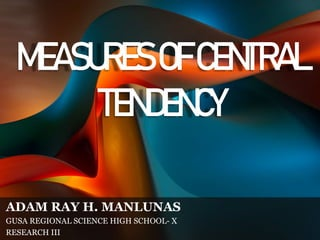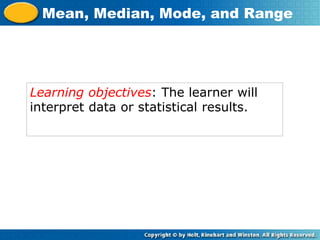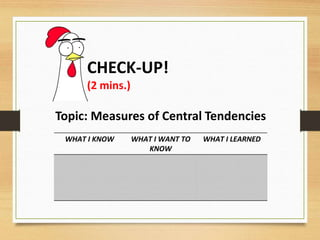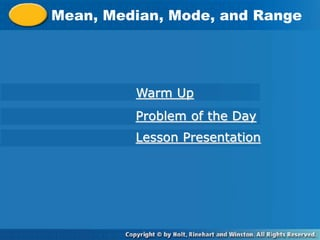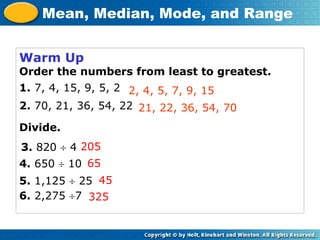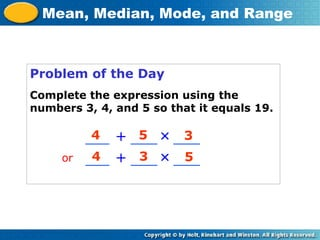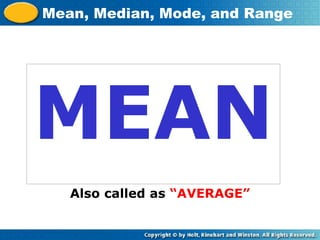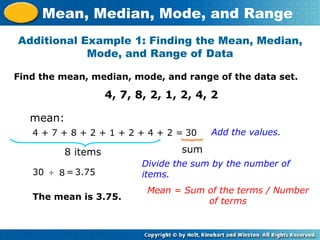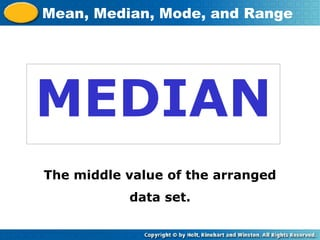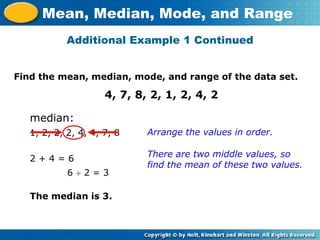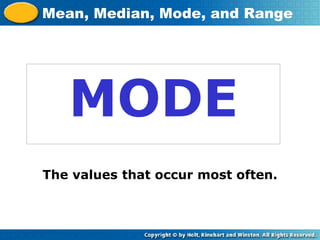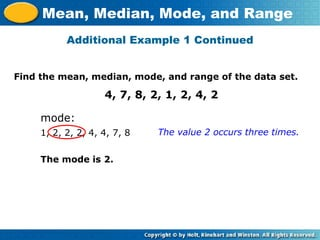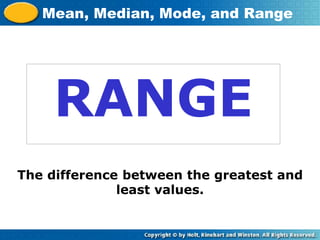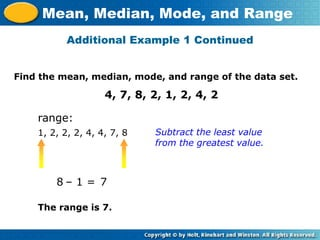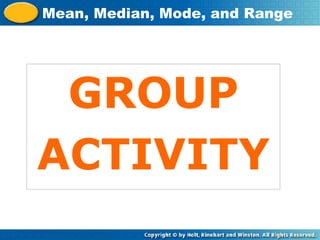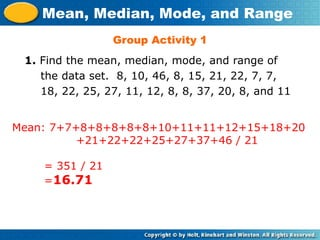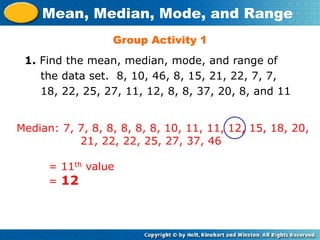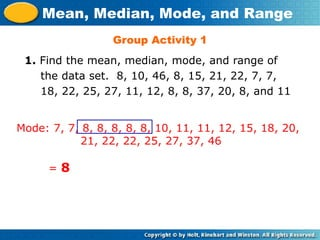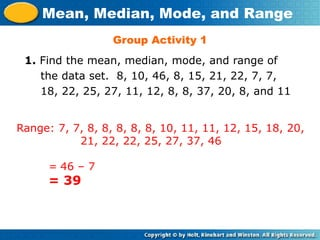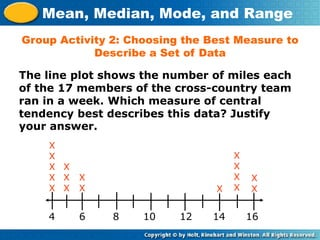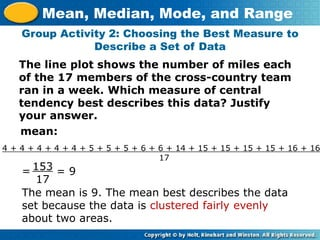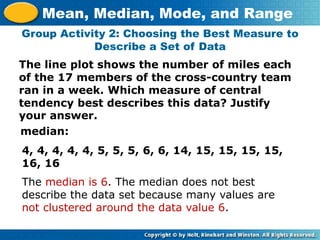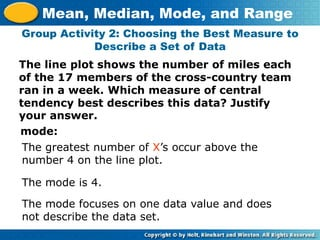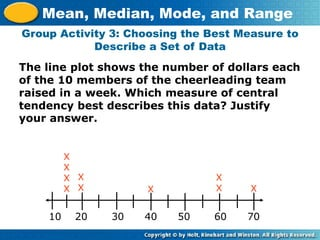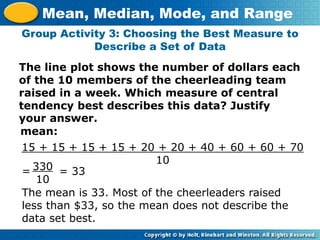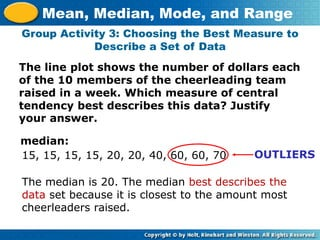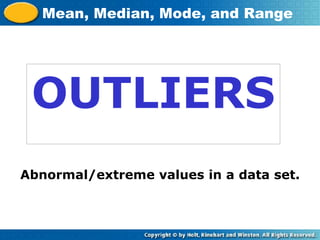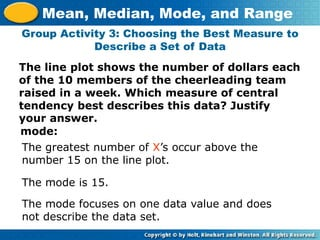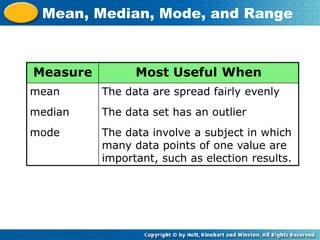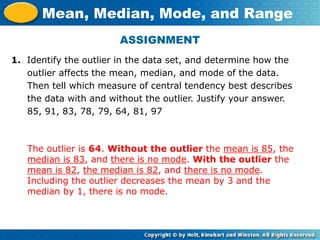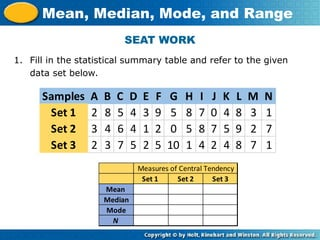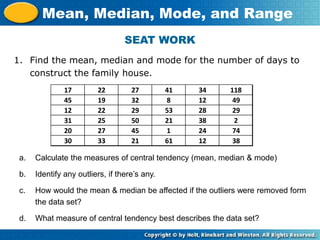1 von 36

### Measures of Central Tendency Final.ppt

• 1. MEASURESOFCENTRAL TENDENCY ADAM RAY H. MANLUNAS GUSA REGIONAL SCIENCE HIGH SCHOOL- X RESEARCH III
• 2. Learning objectives: The learner will interpret data or statistical results. Mean, Median, Mode, and Range
• 3. CHECK-UP! (2 mins.) WHAT I KNOW WHAT I WANT TO KNOW WHAT I LEARNED Topic: Measures of Central Tendencies
• 4. Mean, Median, Mode, and Range Warm Up Problem of the Day Lesson Presentation
• 5. Warm Up Order the numbers from least to greatest. 1. 7, 4, 15, 9, 5, 2 2. 70, 21, 36, 54, 22 Divide. 2, 4, 5, 7, 9, 15 21, 22, 36, 54, 70 205 65 Mean, Median, Mode, and Range 45 325 6. 2,275 7 5. 1,125  25 4. 650  10 3. 820  4
• 6. Problem of the Day Complete the expression using the numbers 3, 4, and 5 so that it equals 19. +  4 5 3 Mean, Median, Mode, and Range 4 3 5 +  or
• 7. MEAN Mean, Median, Mode, and Range Also called as “AVERAGE”
• 8. Find the mean, median, mode, and range of the data set. 4, 7, 8, 2, 1, 2, 4, 2 Additional Example 1: Finding the Mean, Median, Mode, and Range of Data Mean, Median, Mode, and Range mean: Add the values. 4 + 7 + 8 + 2 + 1 + 2 + 4 + 2 = Divide the sum by the number of items. Mean = Sum of the terms / Number of terms 30 30 3.75 8 items The mean is 3.75. sum 8  =
• 9. MEDIAN Mean, Median, Mode, and Range The middle value of the arranged data set.
• 10. Find the mean, median, mode, and range of the data set. 4, 7, 8, 2, 1, 2, 4, 2 Additional Example 1 Continued Mean, Median, Mode, and Range median: Arrange the values in order. 1, 2, 2, 2, 4, 4, 7, 8 There are two middle values, so find the mean of these two values. The median is 3. 2 + 4 = 6 6  2 = 3
• 11. MODE Mean, Median, Mode, and Range The values that occur most often.
• 12. Find the mean, median, mode, and range of the data set. 4, 7, 8, 2, 1, 2, 4, 2 Additional Example 1 Continued Mean, Median, Mode, and Range mode: The value 2 occurs three times. 1, 2, 2, 2, 4, 4, 7, 8 The mode is 2.
• 13. RANGE Mean, Median, Mode, and Range The difference between the greatest and least values.
• 14. Find the mean, median, mode, and range of the data set. 4, 7, 8, 2, 1, 2, 4, 2 Additional Example 1 Continued Mean, Median, Mode, and Range range: Subtract the least value 1, 2, 2, 2, 4, 4, 7, 8 The range is 7. from the greatest value. – 1 = 8 7
• 15. GROUP ACTIVITY Mean, Median, Mode, and Range
• 16. Group Activity 1 1. Find the mean, median, mode, and range of the data set. 8, 10, 46, 8, 15, 21, 22, 7, 7, 18, 22, 25, 27, 11, 12, 8, 8, 37, 20, 8, and 11 Mean, Median, Mode, and Range Mean: 7+7+8+8+8+8+8+10+11+11+12+15+18+20 +21+22+22+25+27+37+46 / 21 = 351 / 21 =16.71
• 17. Group Activity 1 1. Find the mean, median, mode, and range of the data set. 8, 10, 46, 8, 15, 21, 22, 7, 7, 18, 22, 25, 27, 11, 12, 8, 8, 37, 20, 8, and 11 Mean, Median, Mode, and Range Median: 7, 7, 8, 8, 8, 8, 8, 10, 11, 11, 12, 15, 18, 20, 21, 22, 22, 25, 27, 37, 46 = 11th value = 12
• 18. Group Activity 1 1. Find the mean, median, mode, and range of the data set. 8, 10, 46, 8, 15, 21, 22, 7, 7, 18, 22, 25, 27, 11, 12, 8, 8, 37, 20, 8, and 11 Mean, Median, Mode, and Range Mode: 7, 7, 8, 8, 8, 8, 8, 10, 11, 11, 12, 15, 18, 20, 21, 22, 22, 25, 27, 37, 46 = 8
• 19. Group Activity 1 1. Find the mean, median, mode, and range of the data set. 8, 10, 46, 8, 15, 21, 22, 7, 7, 18, 22, 25, 27, 11, 12, 8, 8, 37, 20, 8, and 11 Mean, Median, Mode, and Range Range: 7, 7, 8, 8, 8, 8, 8, 10, 11, 11, 12, 15, 18, 20, 21, 22, 22, 25, 27, 37, 46 = 46 – 7 = 39
• 20. ANY QUESTIONS??
• 21. DON’T FORGET TO HAVE FUN IN LEARNING! THANK YOU!
• 22. The line plot shows the number of miles each of the 17 members of the cross-country team ran in a week. Which measure of central tendency best describes this data? Justify your answer. Group Activity 2: Choosing the Best Measure to Describe a Set of Data Mean, Median, Mode, and Range 4 6 8 10 12 14 16 X X X X X X X X X X X X X X X X X
• 23. The line plot shows the number of miles each of the 17 members of the cross-country team ran in a week. Which measure of central tendency best describes this data? Justify your answer. Group Activity 2: Choosing the Best Measure to Describe a Set of Data Mean, Median, Mode, and Range mean: 4 + 4 + 4 + 4 + 4 + 5 + 5 + 5 + 6 + 6 + 14 + 15 + 15 + 15 + 15 + 16 + 16 17 = 153 17 The mean is 9. The mean best describes the data set because the data is clustered fairly evenly about two areas. = 9
• 24. The line plot shows the number of miles each of the 17 members of the cross-country team ran in a week. Which measure of central tendency best describes this data? Justify your answer. Group Activity 2: Choosing the Best Measure to Describe a Set of Data Mean, Median, Mode, and Range median: The median is 6. The median does not best describe the data set because many values are not clustered around the data value 6. 4, 4, 4, 4, 4, 5, 5, 5, 6, 6, 14, 15, 15, 15, 15, 16, 16
• 25. The line plot shows the number of miles each of the 17 members of the cross-country team ran in a week. Which measure of central tendency best describes this data? Justify your answer. Group Activity 2: Choosing the Best Measure to Describe a Set of Data Mean, Median, Mode, and Range mode: The greatest number of X’s occur above the number 4 on the line plot. The mode is 4. The mode focuses on one data value and does not describe the data set.
• 26. The line plot shows the number of dollars each of the 10 members of the cheerleading team raised in a week. Which measure of central tendency best describes this data? Justify your answer. Group Activity 3: Choosing the Best Measure to Describe a Set of Data Mean, Median, Mode, and Range 10 20 30 40 50 60 70 X X X X X X X X X X
• 27. Group Activity 3: Choosing the Best Measure to Describe a Set of Data Mean, Median, Mode, and Range mean: 15 + 15 + 15 + 15 + 20 + 20 + 40 + 60 + 60 + 70 10 = 330 10 The mean is 33. Most of the cheerleaders raised less than \$33, so the mean does not describe the data set best. = 33 The line plot shows the number of dollars each of the 10 members of the cheerleading team raised in a week. Which measure of central tendency best describes this data? Justify your answer.
• 28. Group Activity 3: Choosing the Best Measure to Describe a Set of Data Mean, Median, Mode, and Range median: The median is 20. The median best describes the data set because it is closest to the amount most cheerleaders raised. 15, 15, 15, 15, 20, 20, 40, 60, 60, 70 The line plot shows the number of dollars each of the 10 members of the cheerleading team raised in a week. Which measure of central tendency best describes this data? Justify your answer. OUTLIERS
• 29. OUTLIERS Mean, Median, Mode, and Range Abnormal/extreme values in a data set.
• 30. Group Activity 3: Choosing the Best Measure to Describe a Set of Data Mean, Median, Mode, and Range mode: The greatest number of X’s occur above the number 15 on the line plot. The mode is 15. The mode focuses on one data value and does not describe the data set. The line plot shows the number of dollars each of the 10 members of the cheerleading team raised in a week. Which measure of central tendency best describes this data? Justify your answer.
• 31. Mean, Median, Mode, and Range Measure Most Useful When mean median mode The data are spread fairly evenly The data set has an outlier The data involve a subject in which many data points of one value are important, such as election results.
• 32. ANY QUESTIONS??
• 33. ASSIGNMENT 1. Identify the outlier in the data set, and determine how the outlier affects the mean, median, and mode of the data. Then tell which measure of central tendency best describes the data with and without the outlier. Justify your answer. 85, 91, 83, 78, 79, 64, 81, 97 The outlier is 64. Without the outlier the mean is 85, the median is 83, and there is no mode. With the outlier the mean is 82, the median is 82, and there is no mode. Including the outlier decreases the mean by 3 and the median by 1, there is no mode. Mean, Median, Mode, and Range
• 34. DON’T FORGET TO HAVE FUN IN LEARNING! THANK YOU!
• 35. SEAT WORK 1. Fill in the statistical summary table and refer to the given data set below. Mean, Median, Mode, and Range Samples A B C D E F G H I J K L M N Set 1 2 8 5 4 3 9 5 8 7 0 4 8 3 1 Set 2 3 4 6 4 1 2 0 5 8 7 5 9 2 7 Set 3 2 3 7 5 2 5 10 1 4 2 4 8 7 1 Set 1 Set 2 Set 3 Mean Median Mode N Measures of Central Tendency
• 36. SEAT WORK 1. Find the mean, median and mode for the number of days to construct the family house. Mean, Median, Mode, and Range 17 22 27 41 34 118 45 19 32 8 12 49 12 22 29 53 28 29 31 25 50 21 38 2 20 27 45 1 24 74 30 33 21 61 12 38 a. Calculate the measures of central tendency (mean, median & mode) b. Identify any outliers, if there’s any. c. How would the mean & median be affected if the outliers were removed form the data set? d. What measure of central tendency best describes the data set?